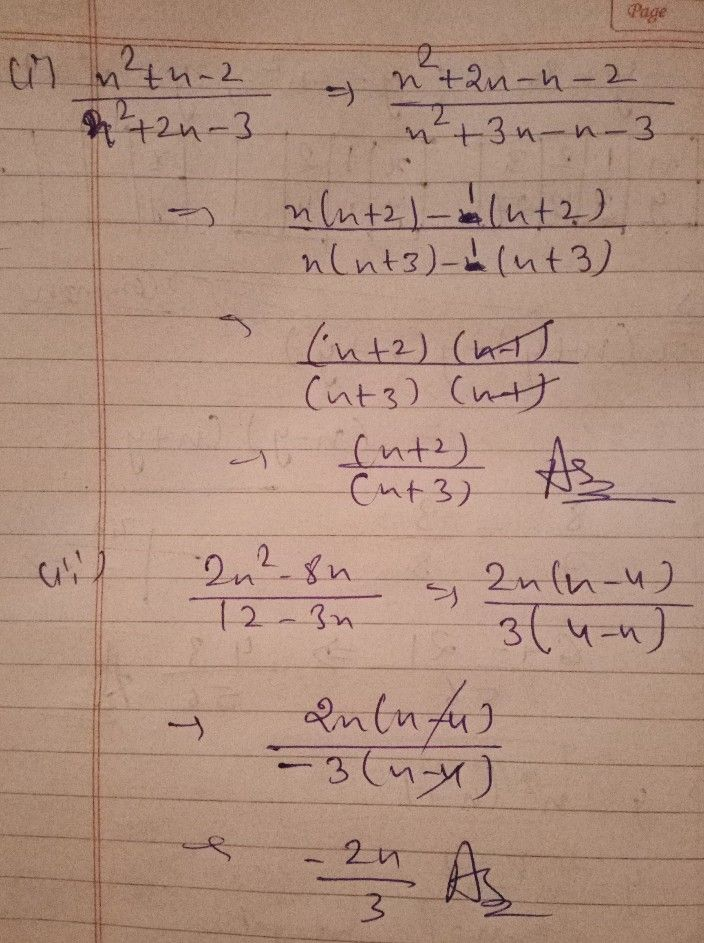Symbol
ProblemWhat $1$ Have Learned What a Wonderful Week Reflect on the topic and activities $y0y$ have $done$ this week by completing the following statements. Write your answers on a separate sheet of paper. This week, I learned about • To reduce a rational algebraic expression to simplest $fom$ there are steps to follow. First then and lastly For instance, in reducing $\dfrac {x^{2}+x-2} {x^{2}+2x-3}$ it should be written $as1$ $\dfrac {x^{2}+x-2} {x^{2}+2x-3}$ %3D It is also possible $t0$ encounter a certain $cas8$ where a factor in the numerator is in opposite sign of a factor in the denominator. In this situation, I need to factor so that the factors will $be$ equivalent. Just like in solving $\dfrac {2x^{2}-8x} {12-3x},$ it should be written as $\dfrac {2x^{2}-8x} {12-3x}$ %3D %3D $ln9$ general, given an expression the rational expression a over the opposite of $ais$ equal $ona≠0$ $tnatis,$ $\dfrac {a} {-9}$ $t0$ Finally, $C$ can say that a rational expression is in simplest form when $1S$ numerator and denominator have
7th-9th grade
Algebra
Search count: 106
SolutionQanda teacher - Nitesh77ok
pls ask if there is any doubt regarding the answer
thank youStudent
Ok na po sir
Thanks po